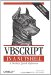# Section C.4. Logical and Bitwise Operators

### C 4 Logical and Bitwise Operators

Logical operators allow you to evaluate one or more expressions and return a logical value. VBA supports six logical operators: And, Or, Not, Eqv, Imp, and Xor. These operators also double as bitwise operators. A bitwise comparison examines each bit position in both expressions and sets or clears the corresponding bit in the result depending upon the operator used. The result of a bitwise operation is a numeric value.

And

Performs logical conjunction; that is, it returns True only if both expression1 and expression2 evaluate to True. If either expression is False, then the result is False. If either expression is Null, then the result is Null. Its syntax is:

`result = expression1 And expression2`

For example:

`If x = 5 And y < 7 Then`

In this case, the code after the If statement will be executed only if the value of x is five and the value of y is less than seven.

As a bitwise operator, And returns 1 if the compared bits in both expressions are 1, and returns 0 in all other cases, as shown in the following table:

Bit in expression1

Bit in expression2

Result

0

0

0

0

1

0

1

0

0

1

1

1

For example, the result of 15 And 179 is 3, as the following binary representation shows:

`00000011 = 00001111 And 10110011`

Or

Performs logical disjunction; that is, if either expression1 or expression2 evaluates to True, or if both expression1 and expression2 evaluate to True, the result is True. Only if neither expression is True does the Or operation return False. If either expression is Null, then the result is also Null. The syntax for the Or operator is:

`result = expression1 Or expression2`

For example:

`If x = 5 Or y < 7 Then`

In this case, the code after the If statement will be executed if the value of x is five or if the value of y is less than seven.

As a bitwise operator, Or returns 0 if the compared bits in both expressions are 0, and returns 1 in all other cases, as shown in the following table:

Bit in expression1

Bit in expression2

Result

0

0

0

0

1

1

1

0

1

1

1

1

For example, the result of 15 Or 179 is 191, as the following binary representation shows:

`10111111 = 00001111 Or 10110011`

Not

Performs logical negation on a single expression; that is, if the expression is True, the Not operator causes it to become False, while if it is False, the operator causes its value to become True. If the expression is Null, though, the result of using the Not operator is still a Null. Its syntax is:

`result = Not expression1`

For example:

`If Not IsNumeric(x) Then`

In this example, the code following the If statement will be executed if IsNumeric returns False, indicating that x is not a value capable of being represented by a number.

As a bitwise operator, Not simply reverses the value of the bit, as shown in the following table:

expression1

Result

0

1

1

0

For example, the result of Not 16 is 239 (or -17, depending on how the high-order bit is interpreted), as the following binary representation shows:

`Not 00010000 = 11101111`

Eqv

Performs logical equivalence; that is, it determines whether the value of two expressions is the same. Eqv returns True when both expressions evaluate to True or both expressions evaluate to False, but it returns False if either expression evaluates to True while the other evaluates to False. Its syntax is:

`result = expression1 Eqv expression2`

As a bitwise operator. Eqv returns 1 if the compared bits in both expressions are the same, and it returns 0 if they are different, as shown in the following table:

Bit in expression1

Bit in expression2

Result

0

0

1

0

1

0

1

0

0

1

1

1

For example, the result of 15 Eqv 179 is 67 (or -189), as the following binary representation shows:

`01000011 = 00001111 Eqv 10110011`

Imp

Performs logical implication, as shown in the following table:

expression1

expression2

Result

True

True

True

True

False

False

True

Null

Null

False

True

True

False

False

True

False

Null

True

Null

True

True

Null

False

Null

Null

Null

Null

Its syntax is:

`result = expression1 Imp expression2 `

As a bitwise operator, Imp returns 1 if the compared bits in both expressions are the same or if expression1 is 1; it returns 0 if the two bits are different and the bit in expression1 is 1, as shown in the following table:

Bit in expression1

Bit in expression2

Result

0

0

1

0

1

1

1

0

0

1

1

1

For example, the result of 15 Imp 179 is 243 (or -13), as the following binary representation shows:

`11110011 = 00001111 Imp 10110011`

Xor

Perform logical exclusion, which is the opposite of Eqv; that is, Xor (an abbreviation for eXclusive OR) determines whether two expressions are different. When both expressions are either True or False, then the result is False. If only one expression is True, the result is True. If either expression is Null, the result is also Null. Its syntax is:

`result = expression1 Xor expression2 `

As a bitwise operator, Xor returns 1 if the bits being compared are different, and returns 0 if they are the same, as shown in the following table:

Bit in expression1

Bit in expression2

Result

0

0

0

0

1

1

1

0

1

1

1

0

For example, the result of 15 Xor 179 is 188, as the following binary representation shows:

`10111100 = 00001111 Xor 10110011`VBScript in a Nutshell, 2nd Edition
ISBN: 0596004885
EAN: 2147483647
Year: 2003
Pages: 335